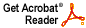# Tangential boundary behavior of functions in Dirichlet-type spaces

Alexander Nagel, Walter Rudin, and Joel H. Shapiro

Annals of Mathematics 116 (1982), 331--360

Abstract: A function holomorphic in the unit disc with its derivative square integrable there has nontangential limits at the boundary of the disc for almost every boundary point, We show here that the approach region for a.e. convergence can be tangential, and can even have exponential order of contact with the boundary a.e.. This result is a special case of the main result, which concerns the existence of tangential limits (through appropriate approach regions forharmonic functions in the upper halfspace R_+^( n+1), whose boundary values correspond to potentials K*f (convolution over R), where f is in L^p, for 1<p<infinity and K is a nonnegative, radial, decreasing, integrable kernel. This result follows in a standard way from the usual weak-type L p estimates for the associated maximal function. However, since the approach regions (and hence the maximal functions) depend on p, the corresponding strong-type L p estimate for p > 1 does not follow simply by interpolation. Rather, it follows from Hansson's capacitary strong-type inequality. With this in hand we establish a trade-off between the order of contact of the approach regions to the boundary and the size of the exceptional sets where tangential convergence may fail, the trade-off being effected by a convolution-factorization of the kernel K. The exceptional sets that arise in this way are of an appropriate capacity zero. The special case of Bessel kernels is treated in detail. Finally, these results and techniques are applied, for example, to studying Carleson measures and the existence of certain holomorphic functions in the halfspace where the imaginary parts of the zeros are prescribed.
Download .pdf file (4.2MB)